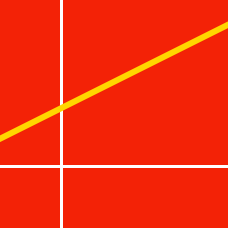Geometry

# Equation of a Line: Level 1 Challenges

Consider the line given by the equation$y=\frac{5}{2}x+30.$ Let $a$ and $b$ be the $x$-intercept and $y$-intercept of this line. What is the value of $a+b$?

Two lines $y-ax=2$ and $x+23y=138$ are perpendicular to each other. What is the value of $a$?

Find the equation of the line which has a slope of $-\frac{3}{4}$ and forms a triangle with the positive coordinate axes such that the triangle has an area of 24 square units.

If three points $A=(k,k+2),$ $B=(0,k-6)$ and $C=(k-4,k)$ all lie on the same line, what is the value of $k?$

The graph of the line $y=ax+b$ passes through the point $(2, 90)$. Additionally, traveling along the line, if $x$ decreases by $2$, then $y$ decreases by $4$. What is the sum of the $x$-intercept and the $y$-intercept?

×

Problem Loading...

Note Loading...

Set Loading...